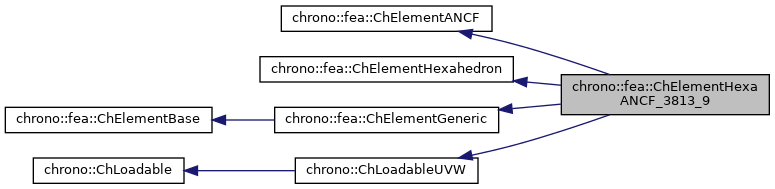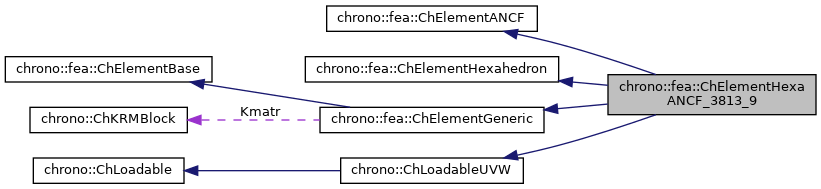chrono::fea::ChElementHexaANCF_3813_9 Class Reference

## Description

Hexahedronal solid element with 8 nodes and a central curvature node.

While technically not an ANCF element, the name is justified because the implementation can use the same ANCF machinery.

#include <ChElementHexaANCF_3813_9.h>

Inheritance diagram for chrono::fea::ChElementHexaANCF_3813_9:[legend]
Collaboration diagram for chrono::fea::ChElementHexaANCF_3813_9:[legend]

## Public Types

enum  StrainFormulation { StrainFormulation::GreenLagrange, StrainFormulation::Hencky }
Strain type calculation. More...

enum  PlasticityFormulation { PlasticityFormulation::J2, PlasticityFormulation::DruckerPrager, PlasticityFormulation::DruckerPrager_Cap }
Plasticity formulation. More...

using ShapeVector = ChMatrixNM< double, 1, 11 >

## Public Member Functions

virtual int GetNnodes () override
Get number of nodes of this element.

virtual int GetNdofs () override
Get the number of coordinates in the field used by the referenced nodes.

virtual int GetNdofs_active () override
Get the number of active coordinates in the field used by the referenced nodes.

virtual int GetNodeNdofs (int n) override
Get the number of coordinates from the n-th node used by this element.

virtual int GetNodeNdofs_active (int n) override
Get the number of active coordinates from the n-th node used by this element.

virtual std::shared_ptr< ChNodeFEAbaseGetNodeN (int n) override
Access the n-th node of this element.

virtual std::shared_ptr< ChNodeFEAxyzGetHexahedronNode (int n) override
Return the specified hexahedron node (0 <= n <= 7).

void SetNodes (std::shared_ptr< ChNodeFEAxyz > node1, std::shared_ptr< ChNodeFEAxyz > node2, std::shared_ptr< ChNodeFEAxyz > node3, std::shared_ptr< ChNodeFEAxyz > node4, std::shared_ptr< ChNodeFEAxyz > node5, std::shared_ptr< ChNodeFEAxyz > node6, std::shared_ptr< ChNodeFEAxyz > node7, std::shared_ptr< ChNodeFEAxyz > node8, std::shared_ptr< ChNodeFEAcurv > nodeC)
Specify the nodes of this element.

std::shared_ptr< ChNodeFEAxyzGetNode1 () const

std::shared_ptr< ChNodeFEAxyzGetNode2 () const

std::shared_ptr< ChNodeFEAxyzGetNode3 () const

std::shared_ptr< ChNodeFEAxyzGetNode4 () const

std::shared_ptr< ChNodeFEAxyzGetNode5 () const

std::shared_ptr< ChNodeFEAxyzGetNode6 () const

std::shared_ptr< ChNodeFEAxyzGetNode7 () const

std::shared_ptr< ChNodeFEAxyzGetNode8 () const

std::shared_ptr< ChNodeFEAcurvGetCentralNode () const

void SetDimensions (const ChVector<> &dims)
DP_Cap parameter. More...

const ChVectorGetDimensions () const
Get the element dimensions (x, y, z directions).

void SetMaterial (std::shared_ptr< ChContinuumElastic > material)
Set the continuum material for this element.

std::shared_ptr< ChContinuumElasticGetMaterial () const
Get a handle to the continuum material used by this element.

Set the structural damping.

void SetStrainFormulation (StrainFormulation model)
Set the strain formulation.

void SetPlasticityFormulation (PlasticityFormulation model)
Set the plasticity formulation.

void SetDPIterationNo (int ItNo)
Set the DP iteration number.

void SetDPVector1 (ChMatrixDynamic< double > vec)
Set the hardening parameter look-up table.

void SetDPVector2 (ChMatrixDynamic< double > vec)

void SetDPVectorSize (int a)

void SetDPCapBeta (double a)
Set DP_Cap parameter.

int GetDPIterationNo () const
Get the DP iteration number.

void SetDPYieldTol (double yieldf_tol)
Set the DP yield function tolerance.

double GetDPYieldTol () const
Get the DP yield function tolerance.

void SetPlasticity (bool val)
Set plasticity.

void SetDruckerPrager (bool val)
Set Drucker-Prager plasticity.

void SetYieldStress (double a)
Set yield stress for yield function.

void SetHardeningSlope (double a)
Set linear isotropic modulus.

void SetFriction (double friction)
Set internal friction angle.

void SetDilatancy (double dilatancy)
Set dilatancy angle.

void SetDPType (int a)
Set Drucker-Prager hardening type.

void SetCCPInitial (ChMatrixNM< double, 9, 8 > mat)
Set initial strain tensor per integration point of the 9-node element.

void ShapeFunctions (ShapeVector &N, double x, double y, double z)
Calculate shape functions and their derivatives. More...

void ShapeFunctionsDerivativeX (ShapeVector &Nx, double x, double y, double z)
Calculate shape function derivative w.r.t. X.

void ShapeFunctionsDerivativeY (ShapeVector &Ny, double x, double y, double z)
Calculate shape function derivative w.r.t. Y.

void ShapeFunctionsDerivativeZ (ShapeVector &Nz, double x, double y, double z)
Calculate shape function derivative w.r.t. Z.

virtual int Get_field_ncoords () override
Number of coordinates in the interpolated field: here the {x,y,z} displacement.

virtual int GetSubBlocks () override
Get the number of DOFs sub-blocks.

virtual unsigned int GetSubBlockOffset (int nblock) override
Get the offset of the specified sub-block of DOFs in global vector.

virtual unsigned int GetSubBlockSize (int nblock) override
Get the size of the specified sub-block of DOFs in global vector.

virtual bool IsSubBlockActive (int nblock) const override
Check if the specified sub-block of DOFs is active.

Get the number of DOFs affected by this element (position part).

Get the number of DOFs affected by this element (speed part).

virtual void LoadableGetStateBlock_x (int block_offset, ChState &mD) override
Get all the DOFs packed in a single vector (position part).

virtual void LoadableGetStateBlock_w (int block_offset, ChStateDelta &mD) override
Get all the DOFs packed in a single vector (speed part).

virtual void LoadableStateIncrement (const unsigned int off_x, ChState &x_new, const ChState &x, const unsigned int off_v, const ChStateDelta &Dv) override
Increment all DOFs using a delta.

virtual void LoadableGetVariables (std::vector< ChVariables * > &mvars) override
Get the pointers to the contained ChLcpVariables, appending to the mvars vector.

virtual void ComputeNF (const double U, const double V, const double W, ChVectorDynamic<> &Qi, double &detJ, const ChVectorDynamic<> &F, ChVectorDynamic<> *state_x, ChVectorDynamic<> *state_w) override
Evaluate N'*F, where N is some type of shape function evaluated at (U,V,W). More...

virtual double GetDensity () override
Return the material density. More...Public Member Functions inherited from chrono::fea::ChElementGeneric
ChKblockGenericKstiffness ()
Access the proxy to stiffness, for sparse solver.

virtual void EleIntLoadResidual_F (ChVectorDynamic<> &R, const double c) override
Add the internal forces (pasted at global nodes offsets) into a global vector R, multiplied by a scaling factor c, as R += forces * c This default implementation is SLIGHTLY INEFFICIENT.

virtual void EleIntLoadResidual_Mv (ChVectorDynamic<> &R, const ChVectorDynamic<> &w, const double c) override
Add the product of element mass M by a vector w (pasted at global nodes offsets) into a global vector R, multiplied by a scaling factor c, as R += M * w * c This default implementation is VERY INEFFICIENT.

virtual void EleIntLoadResidual_F_gravity (ChVectorDynamic<> &R, const ChVector<> &G_acc, const double c) override
Add the contribution of gravity loads, multiplied by a scaling factor c, as: R += M * g * c This default implementation is VERY INEFFICIENT. More...

virtual void InjectKRMmatrices (ChSystemDescriptor &descriptor) override
Tell to a system descriptor that there are item(s) of type ChKblock in this object (for further passing it to a solver)

virtual void KRMmatricesLoad (double Kfactor, double Rfactor, double Mfactor) override
Add the current stiffness K and damping R and mass M matrices in encapsulated ChKblock item(s), if any. More...

virtual void VariablesFbLoadInternalForces (double factor=1.) override
Add the internal forces, expressed as nodal forces, into the encapsulated ChVariables.

virtual void VariablesFbIncrementMq () override
Add M*q (internal masses multiplied current 'qb').Public Member Functions inherited from chrono::fea::ChElementBase
virtual void ComputeNodalMass ()
Compute element's nodal masses.

virtual void EleDoIntegration ()
This is optionally implemented if there is some internal state that requires integration.Public Member Functions inherited from chrono::ChLoadableUVW
virtual bool IsTetrahedronIntegrationNeeded ()
If true, use quadrature over u,v,w in [0..1] range as tetrahedron volumetric coords (with z=1-u-v-w) otherwise use default quadrature over u,v,w in [-1..+1] as box isoparametric coords.

virtual bool IsTrianglePrismIntegrationNeeded ()
If true, use quadrature over u,v in [0..1] range as triangle natural coords (with z=1-u-v), and use linear quadrature over w in [-1..+1], otherwise use default quadrature over u,v,w in [-1..+1] as box isoparametric coords.

## Public Attributes

int m_DP_iterationNo
Maximum number of iteration for DP return mapping.

double m_DP_yield
Tolerance for yield function value (Drucker-Prager)

double m_DPCapBeta

## Friends

class Brick9_Mass

class Brick9_Gravity

class Brick9_Force

class Brick9_JacobianProtected Attributes inherited from chrono::fea::ChElementANCF
int m_element_dof
actual number of degrees of freedom for the element

bool m_full_dof
true if all node variables are active (not fixed)

ChArray< int > m_mapping_dof
indices of active DOFs (set only is some are fixed)Protected Attributes inherited from chrono::fea::ChElementGeneric
ChKblockGeneric Kmatr

## ◆ PlasticityFormulation

 strong

Plasticity formulation.

Enumerator
J2

J2 plasticity (metals)

DruckerPrager

Drucker-Prager plasticity (soil)

DruckerPrager_Cap

Drucker-Prager-Cap plasticity (soil)

## ◆ StrainFormulation

 strong

Strain type calculation.

Enumerator
GreenLagrange

Green-Lagrange strain formulation.

Hencky

Hencky strain.

## ◆ ComputeNF()

 void chrono::fea::ChElementHexaANCF_3813_9::ComputeNF ( const double U, const double V, const double W, ChVectorDynamic<> & Qi, double & detJ, const ChVectorDynamic<> & F, ChVectorDynamic<> * state_x, ChVectorDynamic<> * state_w )
overridevirtual

Evaluate N'*F, where N is some type of shape function evaluated at (U,V,W).

Here, U,V,W are coordinates of the volume, each ranging in -1..+1 F is a load, N'*F is the resulting generalized load Returns also det[J] with J=[dx/du,..], that might be useful in gauss quadrature.

Parameters
 U parametric coordinate in volume V parametric coordinate in volume W parametric coordinate in volume Qi Return result of N'*F here, maybe with offset block_offset detJ Return det[J] here F Input F vector, size is = n.field coords. state_x if != 0, update state (pos. part) to this, then evaluate Q state_w if != 0, update state (speed part) to this, then evaluate Q

## ◆ GetDensity()

 virtual double chrono::fea::ChElementHexaANCF_3813_9::GetDensity ( )
inlineoverridevirtual

Return the material density.

This is needed so that it can be accessed by ChLoaderVolumeGravity.

## ◆ SetDimensions()

 void chrono::fea::ChElementHexaANCF_3813_9::SetDimensions ( const ChVector<> & dims )
inline

DP_Cap parameter.

Set element dimensions (x, y, z directions).

## ◆ ShapeFunctions()

 void chrono::fea::ChElementHexaANCF_3813_9::ShapeFunctions ( ShapeVector & N, double x, double y, double z )

Calculate shape functions and their derivatives.

N = [N1, N2, N3, N4, ...] (1x11 row vector) S = [N1*eye(3), N2*eye(3), N3*eye(3) ,N4*eye(3), ...] (3x11 matrix)

The documentation for this class was generated from the following files:
• /builds/uwsbel/chrono/src/chrono/fea/ChElementHexaANCF_3813_9.h
• /builds/uwsbel/chrono/src/chrono/fea/ChElementHexaANCF_3813_9.cpp